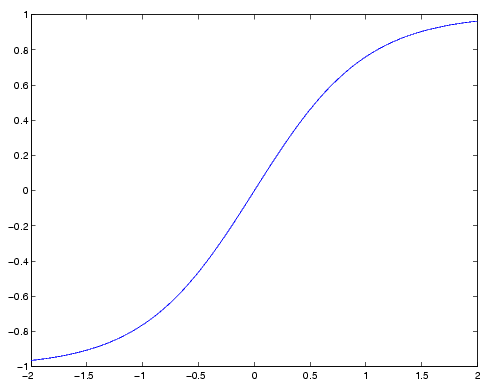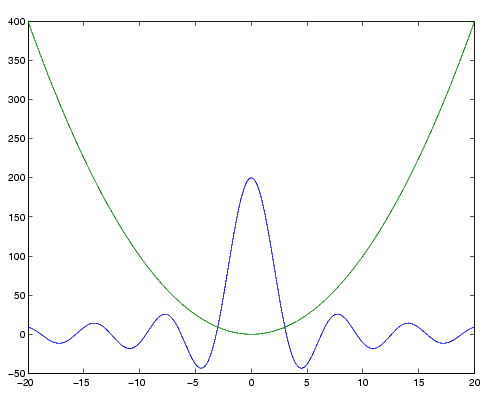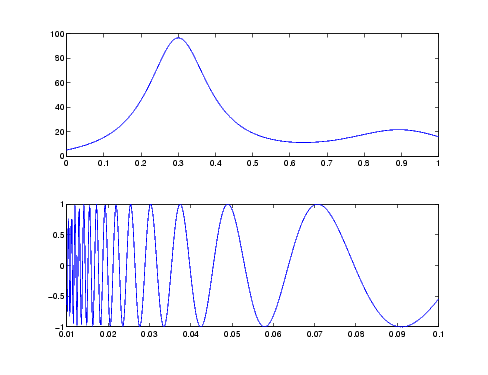MATLAB Function Referencefplot

Plot a function between specified limits

Syntax

• ```fplot(fun,limits)
fplot(fun,limits,LineSpec)
fplot(fun,limits,tol)
fplot(fun,limits,tol,LineSpec)
fplot(fun,limits,n)
fplot(axes_handle,...)
[X,Y] = fplot(fun,limits,...)
```

Description

`fplot` plots a function between specified limits. The function must be of the form y = f(x), where x is a vector whose range specifies the limits, and `y` is a vector the same size as `x` and contains the function's value at the points in x (see the first example). If the function returns more than one value for a given x, then y is a matrix whose columns contain each component of f(x) (see the second example).

```fplot(fun,limits) ``` plots `fun` between the limits specified by `limits`. `limits` is a vector specifying the x-axis limits (`[xmin` `xmax])`, or the x- and y-axes limits, (`[xmin` `xmax` `ymin` `ymax]`).

`fun` must be

• The name of an M-file function
• A string with variable `x` that may be passed to `eval`, such as `'sin(x)'`, `'diric(x,10)'`, or `'[sin(x),cos(x)]'`
• A function handle for an M-file function or an anonymous function (see Function Handles and Anonymous Functions for more information)

The function f(x) must return a row vector for each element of vector x. For example, if f(x) returns [`f1(x),f2(x),f3(x)]` then for input `[x1;x2]` the function should return the matrix

• ```f1(x1) f2(x1) f3(x1)
f1(x2) f2(x2) f3(x2)
```

```fplot(fun,limits,LineSpec) ``` plots `fun` using the line specification `LineSpec`.

```fplot(fun,limits,tol) ``` plots `fun` using the relative error tolerance `tol` (the default is `2e-3`, i.e., 0.2 percent accuracy).

```fplot(fun,limits,tol,LineSpec) ``` plots `fun` using the relative error tolerance `tol` and a line specification that determines line type, marker symbol, and color. See `LineSpec` for more information.

```fplot(fun,limits,n) ``` with `n >= 1` plots the function with a minimum of `n+1` points. The default `n` is `1`. The maximum step size is restricted to be `(1/n)*(xmax-xmin)`.

```fplot(fun,lims,...) ``` accepts combinations of the optional arguments `tol`, `n`, and `LineSpec`, in any order.

```fplot(axes_handle,...) ``` plots into the axes with handle `axes_handle` instead of the current axes (`gca`).

```[X,Y] = fplot(fun,limits,...) ``` returns the abscissas and ordinates for `fun` in `X` and `Y`. No plot is drawn on the screen; however, you can plot the function using `plot(X,Y)`.

Remarks

`fplot` uses adaptive step control to produce a representative graph, concentrating its evaluation in regions where the function's rate of change is the greatest.

Examples

Plot the hyperbolic tangent function from -2 to 2:

• ``````fnch = @tanh;
``````fplot(fnch,[-2 2])
``````

Create an M-file, `myfun`, that returns a two-column matrix:

• ```function Y = myfun(x)
Y(:`,`1) = 200*sin(x(:))./x(:);
Y(:`,`2) = x(:).^2;
```

Create a function handle pointing to `myfun`:

• ```fh = @myfun;
```

Plot the function with the statement

• ```fplot(fh,[-20 20])```

Addition Examples

This example passes function handles to `fplot`, one created from a MATLAB function and the other created from an anonymous function.

• ```hmp = @humps;
subplot(2,1,1);fplot(hmp,[0 1])
sn = @(x) sin(1./x);
subplot(2,1,2);fplot(sn,[.01 .1])```

See Also

`eval`, `ezplot`, `feval`, `LineSpec`, `plot`

Function Plots for related functions

Plotting Mathematical Functions for more examples

© 1994-2005 The MathWorks, Inc.